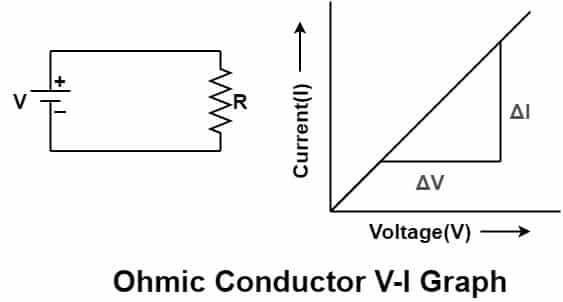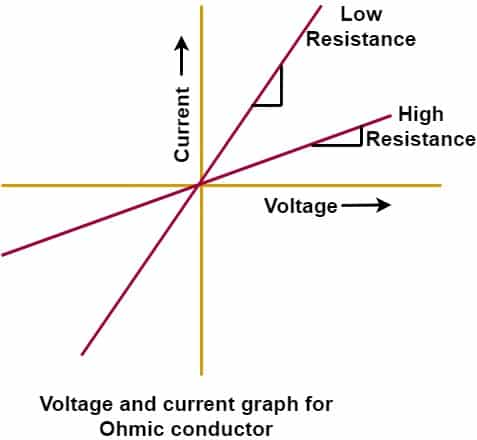# Difference Between Ohmic and Non-Ohmic Conductors

## Ohmic Vs non-Ohmic Conductors

Difference between ohmic and non-ohmic conductor can be found by knowing the relationship between voltage and current. When the voltage is applied across a conductor, the electrons starts moving from high potential to low potential point. The flow of electrons cause flow of electric current in the opposite direction of electrons flow. The magnitude of current flowing in the circuit depends on the applied voltage and the resistance of the circuit. The current remains fairly constant for the  particular magnitude of  applied voltage if the resistance of the circuit remains unchanged. The difference between ohmic and non-ohmic conductor is that an Ohmic conductors have linear relationship between voltage and current, whereas the non-Ohmic conductors do not have linear relationship between voltage and current.

The conductor is whether ohmic or non ohmic can be verified with Ohm’s law. The Ohm’s law states the relationship between voltage, current and resistance.

According to Ohm’s Law

I α V

I = V/ R   ——–(1)
Where,
V = Applied Voltage
I  = Current
R = Resistance of the circuit
Equation(1) can be plotted in a graphical form as given below.

#### V-I Graph for Ohmic ConductorThe slope of the straight line is 1/R. The slope remains constant if the resistance value does not change in a electrical circuit.The slope of the line in positive and negative quadrant is constant. A low resistance component  have more slope line and a higher resistance device  have a much steeper line on the Ohmic conductor graph.#### Definition of Ohmic Conductor

The conductors which follow the above given voltage and current relationship are called the  Ohmic conductor. In other words, the conductors which obey the Ohm’s law are called the Ohmic conductor.

#### Definition of non Ohmic Conductor

The conductors in which the current does not flow in the proportion of the increased voltage is called the non ohmic conductor. In other words, the conductors which do not obey the Ohms’s law are called non-Ohmic conductors.

#### Examples  of  Ohmic Conductors

The example of Ohmic conductor is the resistor. The current flowing through the resistor is directly proportional to the applied voltage and voltage across the resistor and current flowing through it have a linear relationship. The resistance of the conductor depends on the temperature and the resistance increases with an increase in temperature according to temperature coefficient of the resistance. If the current flowing through the resistance increases above its current carrying capacity, the resistance gets increased because of rise of temperature. The increased heat can cause the resistance to become non-Ohmic.

#### Examples of  non ohmic Conductors

The conductor is non-Ohmic if the current flowing through the conductor and the voltage across the device do not have linear relationship. The non-Ohmic conductor does not obey Ohm’s law.The examples of non-Ohmic conductors are filament lamp, semiconductor devices like diodes,transistors, thyristors etc.

#### V-I Graph for  non-ohmic Conductors

In case of the diode the current keep on increasing but the voltage drop across the diode does not increase. That is why diode is non-Ohmic device.The characteristics of the diode is as given below.

In case of the filament lamp, if the voltage is increased the current flowing through the lamp does not increase beyond a particular value. Therefore, the filament lamp is non-Ohmic. Voltage-current characteristics of the incandescent lamp is as given below.

The bulb resistance has a positive temperature coefficient. The resistance of the bulb increase with an increase in the temperature. The filament resistance changes with increase in the current due to temperature increase. The filament resistance does not remain constant with an increase of current. Therefore, the incandescent/filament lamp does not obey the Ohm’s law and filament lamp is a non ohmic.

Related Posts:

1. Why is Diode Non-Ohmic Semiconductor Device?
2. Diode Current Equation